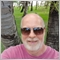# Max bars in chart and how it affects rates_total, prev_calculated and the time series arrays?55

There is a setting in the client terminal to limit the number of bars on the chart. Mine is currently set to 100,000. How does this affect rates_total, prev_calculated and the time series arrays passed to OnCalculate?

For most on the calls, rates_total and the time series arrays will grow in size as new bars are added. And prev_calculated will have the same value as returned from the previous call to OnCalculate. But, what happens when the client terminal has reached the max bars limit or some value close to the limit? On the next call of OnCalculate will rates_total now become a much smaller value, will the time series arrays have their data shifted down meaning the oldest data is lost, and what will the value of prev_calculated become?

The answers to these questions appear to be missing from the documentation.20105

1. Max on chart affects the initial bar count and thus rates_total.
2. On MT4: it does not limit the bar count. Rates_total does not become smaller, ever.
3. Has no effect for prev_calculated.
1. Terminal starts.
2. Indicators/EAs are loaded. Static and globally declared variables are initialized. (Do not depend on a specific order.)
3. OnInit is called.
4. For indicators OnCalculate is called with any existing history.
5. Human may have to enter password, connection to server begins.
6. New history is received, OnCalculate called again.
7. New tick is received, OnCalculate/OnTick is called. Now TickValue, TimeCurrent, account information and prices are valid.55

If I understand you correctly on a 1 minute timeframe there will be 1440 new bars added each day, meaning rates_total and the time series arrays will increase by the same amount. Are you suggesting that there is no limit and the size of the arrays will continue to grow. This does not seem likely, at some point the system must reset rates_total and the array sizes?20105

When in doubt, test it. Set MBOC to 500 and open a M1. You keep stating assumptions — I keep stating you're wrong.38391

William Roeder:
1. Max on chart affects the initial bar count and thus rates_total.
2. It does not limit the bar count. Rates_total does not become smaller, ever.
3. Has no effect for prev_calculated.

You are wrong.

For MT5 (I didn't check MT4), when the total number of bars (rates_total) reached the limit, every X bars (I didn't investigate to find the exact rule(s), it could be every day so 1440 bars on M1, and it could also depend on the value of Max bars), the oldest bars are removed, rates_total become smaller and prev_calculated=0, which should lead to a complete recalculation of the indicator.55

Alain Verleyen:

You are wrong.

For MT5 (I didn't check MT4), when the total number of bars (rates_total) reached the limit, every X bars (I didn't investigate to find the exact rule(s), it could be every day so 1440 bars on M1, and it could also depend on the value of Max bars), the oldest bars are removed, rates_total become smaller and prev_calculated=0, which should lead to a complete recalculation of the indicator.

Thank you Alain! I completely agree, and is exactly what I have since discovered - the idea of rates_total and the size of the arrays increasing forever is dumb. This is what I have added to the start of my OnCalculate():

if (rates_total < prev_calculated)

{

return 0; // on next call prev_calculated will be 0

}

if (prev_calculated == 0)

{

// initialisation code here

}